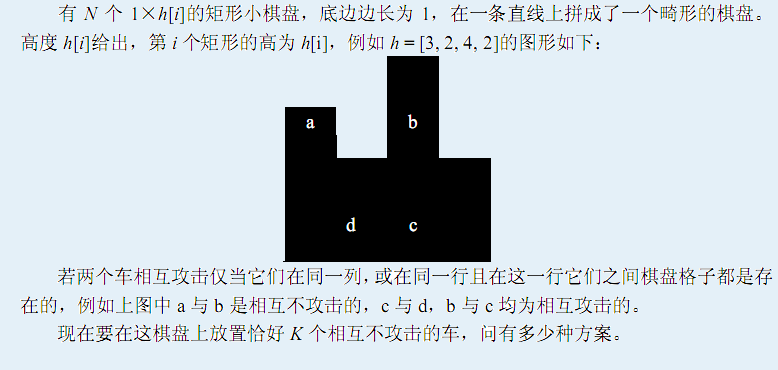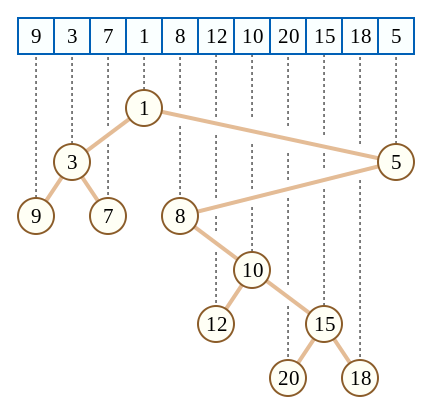# 【BZOJ】P2616 SPOJ PERIODNI

## 笛卡尔树+树形dp1.二叉树

2.若只看权值，则其每个点在权值上形成一个

3.若只看下标，则其每个点在下标上形成一个二叉搜索树1.如果$x>top$，则将top的右儿子设置为x

2.如果$x \le top$，则将top弹出，当$top>x$或者栈为空为止，记最后一个弹出的数为la，则将la设置为x的左二子；若栈不为空，则将x设置为top的右儿子

for(int i=1; i<=n; i++) {
int res=-1;
while(head<=tail&&A[i]<=A[Q[tail]])res=Q[tail--];
if(res!=-1) {
if(rt==res)rt=i;
ch[i]=res;
}
if(head<=tail)ch[Q[tail]]=i;
Q[++tail]=i;
}


$dp[x][i]$表示在以x为根的子树中，放了i个棋子且放的位置大于$h[fa]$的方案数，其中fa表示x的父亲

dp[x]=1;
size[x]=1;
for(int i=0; i<=1; i++) {
int t=ch[x][i];
if(t==0)continue;
DFS(t,x);
for(int i=min(K,size[x]); i>=0; i--) {
for(int j=min(K,size[t]); j>=1; j--) {
if(i+j>K)continue;
if(i+j==0)continue;
dp[x][i+j]=(dp[x][i+j]+dp[x][i]*dp[t][j]%MOD)%MOD;
}
}
size[x]+=size[t];
}


#include<bits/stdc++.h>
#define int long long
using namespace std;
const int MOD=1000000007;
int n,K,rt,head,tail,A,ch,Q,dp,size,frac,inv,infr;
int Quick_Pow(int a,int p){
int res=1;
while(p){
if(p&1)res=res*a%MOD;
a=a*a%MOD;
p>>=1;
}
return res;
}
int C(int x,int y){
if(x<y)return 0;
return frac[x]*infr[y]%MOD*infr[x-y]%MOD;
}
void DFS(int x,int fa){
dp[x]=1;
size[x]=1;
for(int i=0;i<=1;i++){
int t=ch[x][i];
if(t==0)continue;
DFS(t,x);
for(int i=min(K,size[x]);i>=0;i--){
for(int j=min(K,size[t]);j>=1;j--){
if(i+j>K)continue;
if(i+j==0)continue;
dp[x][i+j]=(dp[x][i+j]+dp[x][i]*dp[t][j]%MOD)%MOD;
}
}
size[x]+=size[t];
}
int cha=A[x]-A[fa];
for(int i=min(size[x],K);i>=0;i--){
for(int j=size[x]-i;j>=1;j--){
if(i+j>K)continue;
if(i+j==0)continue;
dp[x][i+j]=(dp[x][i+j]+dp[x][i]*C(size[x]-i,j)%MOD*C(cha,j)%MOD*frac[j]%MOD)%MOD;
}
}
}
void init(){
frac=infr=1;
for(int i=1;i<=1000010;i++)inv[i]=Quick_Pow(i,MOD-2);
for(int i=1;i<=1000010;i++)frac[i]=frac[i-1]*i%MOD,infr[i]=infr[i-1]*inv[i]%MOD;
}
signed main() {
init();
scanf("%lld %lld",&n,&K);
rt=1,head=1,tail=0;
for(int i=1; i<=n; i++)scanf("%lld",&A[i]);
for(int i=1; i<=n; i++) {
int res=-1;
while(head<=tail&&A[i]<=A[Q[tail]])res=Q[tail--];
if(res!=-1) {
if(rt==res)rt=i;
ch[i]=res;
}
if(head<=tail)ch[Q[tail]]=i;
Q[++tail]=i;
}
DFS(rt,0);
printf("%lld",dp[rt][K]);
return 0;
}

posted @ 2019-09-10 12:52  TieT  阅读(191)  评论(0编辑  收藏  举报